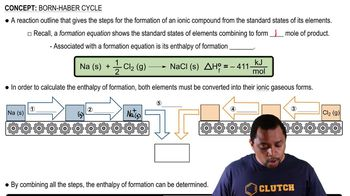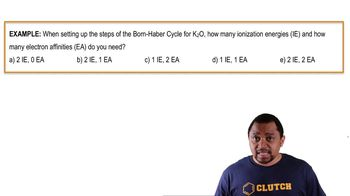Start typing, then use the up and down arrows to select an option from the list.
3:56 minutes
Problem 48
Textbook Question

# Use the Born–Haber cycle and data from Appendix IIB and Table 9.3 to calculate the lattice energy of CaO. (ΔHsub for calcium is 178 kJ>mol; IE1 and IE2 for calcium are 590 kJ>mol and 1145 kJ>mol, respectively; EA1 and EA2 for O are -141 kJ>mol and 744 kJ>mol, respectively.)Verified Solution
This video solution was recommended by our tutors as helpful for the problem above.
512views
1